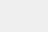# python共现矩阵的实现代码

小编写这篇文章的主要目的，主要是给大家介绍，关于python中，共现矩阵代码实现方式的问题，下面就给大家进行详细的解答。

python共现矩阵实现

最近在学习python词库的可视化，其中有一个依据共现矩阵制作的可视化，感觉十分炫酷，便以此复刻。项目背景

本人利用搜索引擎爬虫，以此用来获取各大博客网站的文章，在进行jieba分词，得到每篇文章的关键词，对这些关键词进行共现矩阵的可视化。

什么是共现矩阵

比如我们有两句话：

`ls=['我永远喜欢小泽玛利亚','小泽玛利亚又出新作了']`

在jieba分词下我们可以得到如下效果：我们就可以构建一个以关键词的共现矩阵：

```　　['','我','永远','喜欢','小泽','玛利亚,'又','出','新作','了']
['我',0,1,1,1,1,0,0,0,0]
['永远',1,0,1,1,1,0,0,0,0]
['喜欢'1,1,0,1,1,0,0,0,0]
['小泽',1,1,1,0,1,1,1,1,1]
['玛利亚',1,1,1,1,0,1,1,1,1]
['又',0,0,0,1,1,0,1,1,1]
['出',0,0,0,1,1,1,0,1,1]
['新作',0,0,0,1,1,1,1,0,1]
['了',0,0,0,1,1,1,1,1,0]]```

解释一下，“我永远喜欢小泽玛利亚”，这一句话中，“我”和“永远”共同出现了一次，在共现矩阵对应的[i][j]和[j][i]上+1，并依次类推。

基于这个原因，我们可以发现，共现矩阵的特点是：

共现矩阵的为空。

共现矩阵的第一行第一列是关键词。

对角线全为0。

共现矩阵其实是一个对称矩阵。

当然，在实际的操作中，这些关键词是需要经过清洗的，这样的可视化才干净。

共现矩阵的构建思路

每篇文章关键词的二维数组data_array。

所有关键词的集合set_word。

建立关键词长度+1的矩阵matrix。

赋值矩阵的第一行与第一列为关键词。

设置矩阵对角线为0。

遍历formated_data，让取出的行关键词和取出的列关键词进行组合，共现则+1。

共现矩阵的代码实现

```　#coding:utf-8
import numpy as np
import pandas as pd
import jieba.analyse
import os
#获取关键词
def Get_file_keywords(dir):
data_array=[]#每篇文章关键词的二维数组
set_word=[]#所有关键词的集合
try:
fo=open('dic_test.txt','w+',encoding='UTF-8')
for home,dirs,files in os.walk(dir):#遍历文件夹下的每篇文章
for filename in files:
fullname=os.path.join(home,filename)
f=open(fullname,'r',encoding='UTF-8')
words="".join(jieba.analyse.extract_tags(sentence=sentence,topK=30,withWeight=False,
allowPOS=('n')))#TF-IDF分词
words=words.split('')
data_array.append(words)
for word in words:
if word not in set_word:
set_word.append(word)
set_word=list(set(set_word))#所有关键词的集合
return data_array,set_word
except Exception as reason:
print('出现错误：',reason)
return data_array,set_word
#初始化矩阵
def build_matirx(set_word):
edge=len(set_word)+1#建立矩阵，矩阵的高度和宽度为关键词集合的长度+1
'''matrix=np.zeros((edge,edge),dtype=str)'''#另一种初始化方法
matrix=[[''for j in range(edge)]for i in range(edge)]#初始化矩阵
matrix[1:]=np.array(set_word)
matrix=list(map(list,zip(*matrix)))
matrix[1:]=np.array(set_word)#赋值矩阵的第一行与第一列
return matrix
#计算各个关键词的共现次数
def count_matrix(matrix,formated_data):
for row in range(1,len(matrix)):
#遍历矩阵第一行，跳过下标为0的元素
for col in range(1,len(matrix)):```

共现矩阵(共词矩阵)计算

共现矩阵(共词矩阵)

统计文本中两两词组之间共同出现的次数，以此来描述词组间的亲密度

code(我这里求的对角线元素为该字段在文本中出现的总次数)：

```　　import pandas as pd
def gx_matrix(vol_li):
#整合一下，输入是df列，输出直接是矩阵
names=locals()
all_col0=[]#用来后续求所有字段的集合
for row in vol_li:
all_col0+=row
for each in row:#对每行的元素进行处理，存在该字段字典的话，再进行后续判断，否则创造该字段字典
try:
for each1 in row:#对已存在字典，循环该行每个元素，存在则在已有次数上加一，第一次出现创建键值对“字段：1”
try:
names['dic_'+each][each1]=names['dic_'+each][each1]+1#尝试，一起出现过的话，直接加1
except:
names['dic_'+each][each1]=1#没有的话，第一次加1
except:
names['dic_'+each]=dict.fromkeys(row,1)#字段首次出现，创造字典
#根据生成的计数字典生成矩阵
all_col=list(set(all_col0))#所有的字段（所有动物的集合）
all_col.sort(reverse=False)#给定词汇列表排序排序，为了和生成空矩阵的横向列名一致
df_final0=pd.DataFrame(columns=all_col)#生成空矩阵
for each in all_col:#空矩阵中每列，存在给字段字典，转为一列存入矩阵，否则先创造全为零的字典，再填充进矩阵
try:
temp=pd.DataFrame(names['dic_'+each],index=[each])
except:
names['dic_'+each]=dict.fromkeys(all_col,0)
temp=pd.DataFrame(names['dic_'+each],index=[each])
df_final0=pd.concat([df_final0,temp])#拼接
df_final=df_final0.fillna(0)
return df_final
if __name__=='__main__':
temp1=['狗','狮子','孔雀','猪']
temp2=['大象','狮子','老虎','猪']
temp3=['大象','北极熊','老虎','猪']
temp4=['大象','狗','老虎','小鸡']
temp5=['狐狸','狮子','老虎','猪']
temp_all=[temp2,temp1,temp3,temp4,temp5]
vol_li=pd.Series(temp_all)
df_matrix=gx_matrix(vol_li)
print(df_matrix)```

输入是整成这个样子的series最后转为df求出每个字段与各字段的出现次数的字典补充一点

这里如果用大象所在列，除以大象出现的次数，比值高的，表明两者一起出现的次数多，如果这列比值中，有两个元素a和b的比值均大于0.8(也不一定是0.8啦)，就是均比较高，则说明a和b和大象三个一起出现的次数多！！！

即可以求出文本中经常一起出现的词组搭配，比如这里的第二列，大象一共出现3次，与老虎出现3次，与猪出现2次，则可以推导出大象，老虎，猪一起出现的概率较高。

也可以把出现总次数拎出来，放在最后一列，则代码为：

#计算每个字段的出现次数，并列为最后一行

df_final['all_times']=''

for each in df_final0.columns:

df_final['all_times'].loc[each]=df_final0.loc[each,each]

放在上述代码df_final=df_final0.fillna(0)的后面即可

结果为